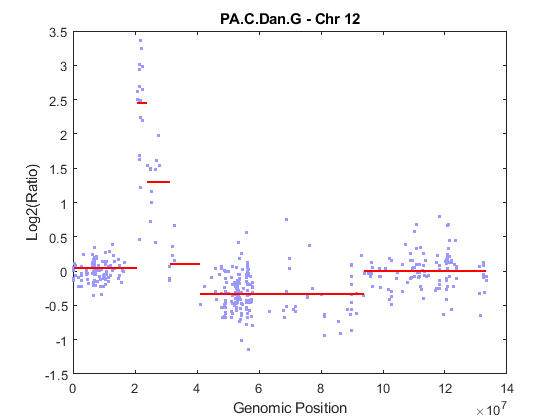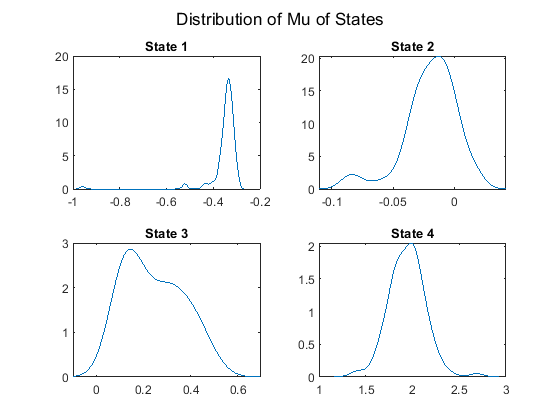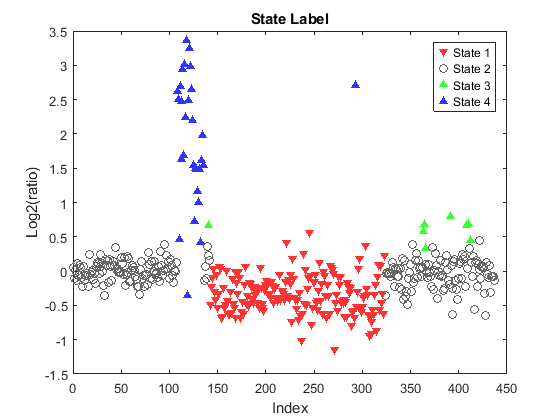Documentation

## Analyzing Array-Based CGH Data Using Bayesian Hidden Markov Modeling

This example shows how to use a Bayesian hidden Markov model (HMM) technique to identify copy number alteration in array-based comparative genomic hybridization (CGH) data.

### Introduction

Array-based CGH is a high-throughput technique to measure DNA copy number change across the genome. The DNA fragments or "clones" of test and reference samples are hybridized to mapped array fragments. Log2 intensity ratios of test to reference provide useful information about genome-wide profiles in copy number. In an ideal situation, the log2 ratio of normal (copy-neutral) clones is log2(2/2) = 0, single copy losses is log2(1/2) = -1, and single copy gains is log2(3/2) = 0.58. Multiple copy gains or amplifications would have values of log2(4/2), log2(5/2),.... Loss of both copies, or a deletion would correspond to the value of -inf. In real applications, even after accounting for measurement error, the log2 ratios differ considerably from the theoretical values. The ratios typically shrink towards zero. One main factor is contamination of the tumor samples with normal cells. There is also a dependence between the intensity ratios of neighboring clones. These issues necessitate the use of efficient statistical algorithms characterizing the genomic profiles.

### Bayesian HMM

Guha et al., (2006) proposed a Bayesian statistical approach depending on a hidden Markov model (HMM) for analyzing array CGH data. The hidden Markov model accounts for the dependence between neighboring clones. The intensity ratios are generated by hidden copy number states. Bayesian learning is used to identify genome-wide changes in copy number from the data. Posterior inferences are made about the copy number gains and losses.

In this Bayesian HMM algorithm, there are four states, defined as copy number loss state (1), copy number neutral state (2), single copy gain state (3), and amplification (multiple gain) state (4). A copy number state is associated with each clone. The normalized log2 ratios are assumed to be distributed as

``

The mu is a unknown parameter for each state with this constraint:

``

The priors for mean copy number changes are:

``
``
``
``

Guha et al., (2006) also described an Metropolis-within-Gibbs algorithm to generate posterior samples. The MCMC algorithm is independently run for each chromosome to generate an MCMC sample for the chromosome parameters. The starting values of the parameters are generated from the priors. The generated copy number states represent draws from the marginal posterior of interest, For each MCMC draw, the generated states are inspected and classified as focal ablations, transition points, amplifications, outliers and whole chromosomal changes.

In this example, you will apply the Bayesian HMM algorithm to analyze the array CGH profiles of some pancreatic cancer samples .

### Loading the Data

The data in this example is the array CGH profiles of 24 pancreatic adenocarcinoma cell lines and 13 primary tumor specimens from Alguirre et al.,(2004). Labeled DNA fragments were hybridized to Agilent® human cDNA microarrays containing 14,160 cDNA clones. About 9,420 clones have unique map positions with a median interval between mapped elements of 100.1 kb. More details of the data and experiment can be found in . For convenience, the data has already been normalized and the log2 based intensity ratios are provided by the MAT file `pancrea_oligocgh.mat`.

You will apply the Bayesian HMM algorithm to analyze chromosome 12 of sample 6 of the pancreatic adenocarcinoma data, and compare the results with the segments found by the circular binary segmentation (CBS) algorithm of Olshen et al.,(2004).

Load the MAT file containing the log2 intensity ratios and mapped genomic positions of the 37 samples.

```load pancrea_oligocgh pancrea_data ```
```pancrea_data = struct with fields: Sample: {37x1 cell} Chromosome: [13446x1 int8] GenomicPosition: [13446x1 int32] Log2Ratio: [13446x37 double] Log2RatioMed: [13446x37 double] Log2RatioSeg: [13446x37 double] CloneIDs: [13446x1 int32] ```

Specify the chromosome number and sample to analyze.

```sampleIndex = 6; chromID = 12; sample = pancrea_data.Sample{sampleIndex} ```
```sample = 'PA.C.Dan.G' ```

Load and plot the log2 ratio data of chromosome 12 from sample PA.C.Dan.G.

```idx = pancrea_data.Chromosome == chromID; X = double(pancrea_data.GenomicPosition(idx)); Y = pancrea_data.Log2Ratio(idx, sampleIndex); % Remove NaN data points idx = ~isnan(Y); X = X(idx); Y = Y(idx); % Plot the data figure; plot(X, Y, '.', 'color', [0.6 0.6 1]) ylims = [-1.5, 3.5]; ylim(gca, ylims) title(sprintf('%s - Chromosome %d', sample, chromID)) xlabel('Genomic Position'); ylabel('Log2(Ratio)') ```Number of clones on chromosome 12 to be analyzed

```N = numel(Y) ```
```N = 437 ```

### Performing Circular Binary Segmentation

You can start the analysis by performing chromosomal segmentation using the CBS algorithm , which is implemented in the `cghcbs` function. The process will take several seconds. You can view the plot of the segment means over the original data by specifying the `SHOWPLOT` parameter. Note: You can type `doc cghcbs` for more details on this function.

```PS = cghcbs(pancrea_data, 'SampleInd', sampleIndex, ... 'Chromosome', chromID, 'ShowPlot', chromID); ylim(gca, ylims) ```
```Analyzing: PA.C.Dan.G. Current chromosome 12 ```As shown in the figure, the CBS procedure declared the set of high intensity ratios as two separate segments. The CBS procedure also found a region with copy number losses.

### Initializing Parameters

The Bayesian HMM approach uses a Metropolis-within-Gibbs algorithm to generate posterior samples of the parameters . The model parameters are grouped into four blocks. The algorithm iteratively generates each of the four blocks conditional on the remaining blocks and the data.

To analyze the data with the Bayesian HMM algorithm, you need to initialize the parameters. More details on prior parameters can be found in references  and .

Initialize the state of the random number generator to ensure that the figures generated by these command match the figures in the HTML version of this example.

```rng('default'); ```

Define the number of states

```NS = 4; ```

Define the number of MCMC iterations

```NMC = 100; ```

Determine the hyperparameters of the prior distributions for the four states.

```mus_hyper = [-1, 0, 0.58, 1]; taus_hyper = [1, 1, 1, 2]; ```

Set the parameter epsilon which determines the constrains of the means.

```eps = 0.1; ```

Set the bounds of the prior means of each state.

```mu_low_bounds = [-Inf, -eps, eps, 0.58]; mu_up_bounds = [-eps, eps, 0.58, Inf]; ```

Guha et al., (2006) assumes the inverse of the prior error variances (sigma^2) as gamma distributions with lower bounds of 0.41 for states 1, 2 and 3. Set the scale parameters for the gamma distributions for each state.

```sg_alpha = [1 1 1 1]; sg_beta = [1, 1, 1, 1]; sg_bounds = [0.41 0.41 0.41 1]; ```

Define a variable `states` to store the copy number state sequences of the clones for each MCMC iteration.

```states = zeros(N, NMC); ```

Define a variable `st_counts` to hold the state transition counts for each copy number state.

```st_counts = zeros(NS, NS); ```

### Determining the Prior Distributions

The MCMC iteration starts at

```iloop = 1; ```

Determine sigmas for the four states by sampling from gamma distribution with prior scale parameter alpha and beta.

```sigmas = zeros(NS, NMC); for i = 1:NS sigmas(i, iloop) = acghhmmsample('gamma', sg_alpha(i), sg_beta(i), sg_bounds(i)); end ```

Determine means for the four states by sampling from truncated normal distribution between the lower and upper bounds of the means. Note: The fourth state lower bound will be determined by the third state.

```mus = zeros(NS, NMC); for i = 1:NS if i == 4 mu_low_bounds(4) = mus(3,iloop) + 3*sigmas(3,iloop); end mus(i, iloop) = acghhmmsample('normal', mus_hyper(i), taus_hyper(i),... mu_low_bounds(i), mu_up_bounds(i)); end ```

Assume independent Dirichlet priors for the rows of the stochastic 4x4 transition probability matrix . Generate the stochastic prior transition matrix A from the Dirichlet distributions.

```a = ones(NS, NS); A = acghhmmsample('dirichlet', a, NS); ```

The transition matrix has a unique stationary distribution. The stationary distribution PI is an eigenvector of the transition matrix associated with the eigenvalue 1.

```PI =@(x, n) (ones(1,n)/(eye(n) -x + ones(n)))'; ```

Generate the prior stationary distribution PI.

```Pi = PI(A, NS); ```

Generate the initial emission matrix B

```B = zeros(NS, N); for i = 1:NS B(i,:) = normpdf(Y, mus(i,iloop), sigmas(i,iloop)); end ```

Decode initial hidden states of the clones using a stochastic forward-backward algorithm .

```states(:, iloop) = acghhmmfb(Pi, A, B); ```

### Generating Posterior Samples

For each MCMC iteration, the four blocks of parameters are generated as follows : Update block B1 using a Metropolis-Hastings step to generate the transition matrix, update block B2 the copy number states using a stochastic forward propagate backward sampling algorithm, update block B3 by computing the mus, and update block B4 to generate sigmas.

```for iloop = 2:NMC % Compute the number of transitions from state i to state j for i =1:NS for j = 1:NS st_counts(i, j) = sum((states(1:N-1, iloop-1) == i) .* (states(2:N, iloop-1) == j)); end end % Updating block B1 % Generate the transition matrix from the Dirichlet distributions C = acghhmmsample('dirichlet', st_counts + 1, NS); % Compute the state probabilities under stationary distribution of a % given transition matrix C. PiC = PI(C, NS); % Compute the accepting probability using a Metropolis-Hastings step beta = min([1, exp(log(PiC(states(1, iloop-1))) - log(Pi(states(1, iloop-1))))]); if rand < beta A = C; Pi = PiC; end % Updating block B2 % Generate copy number states using Forward propagate, backward sampling . states(:, iloop) = acghhmmfb(Pi, A, B); % Updating blocks B3 and B4 for i = 1:NS idx_s = states(:, iloop) == i; num_states = sum(idx_s); % If state i is not observed, then draw from its prior parameters if num_states == 0 mus(i, iloop) = acghhmmsample('normal', mus_hyper(i),... taus_hyper(i), mu_low_bounds(i), mu_up_bounds(i)); sigmas(i, iloop)= acghhmmsample('gamma', sg_alpha(i),... sg_beta(i), sg_bounds(i)); else Y_avg = mean(Y(idx_s)); theta_prec = 1/taus_hyper(i)^2 + num_states/sigmas(i,iloop-1)^2; weight_means = (mus_hyper(i)/(taus_hyper(i)^2) +... Y_avg * num_states/(sigmas(i, iloop-1)^2))/theta_prec; % Compute mus - B3 mus(i, iloop) = acghhmmsample('normal', weight_means, ... 1/sqrt(theta_prec), mu_low_bounds(i), mu_up_bounds(i)); % Compute sigmas - B4 Y_v = sum((Y(idx_s) - mus(i, iloop)).^2); sigmas(i, iloop) = acghhmmsample('gamma', sg_alpha(i)+num_states/2,... sg_beta(i)+Y_v/2, sg_bounds(i)); end % Update the emission matrix with new mus and sigmas. B(i,:) = normpdf(Y, mus(i,iloop),sigmas(i,iloop)); end end ```

Plot the posterior mean mu distributions of the four states.

```figure; for j = 1:NS subplot(2,2,j) ksdensity(mus(j,:)); title(sprintf('State %d', j)) end suptitle('Distribution of Mu of States'); hold off; ```Plot the posterior sigma distributions of the four states.

```figure; for j = 1:NS subplot(2,2,j) ksdensity(sigmas(j,:)); title(sprintf('State %d', j)) end suptitle('Distribution of Sigma of States'); hold off; ```### Posterior Inference

Draw a state label for each clone from the MCMC sampling and compute the posterior probabilities of each state.

```clone_states = zeros(1, N); state_prob = zeros(NS, N); state_count = zeros(NS, N); for i = 1:N % for each clone state = states(i, :); for j=1:NS state_count(j, i) = sum(state == j); end selState = find(state_count(:,i) == max(state_count(:,i))); if length(selState) > 1 if i ~= 1 clone_states(i) = clone_states(i-1); else clone_states(i) = min(selState); end else clone_states(i) = selState; end state_prob(:, i) = state_count(:,i)/NMC; end clone_states = clone_states'; ```

Plot the state label for each clone on chromosome 12 of sample PA.C.Dan.G.

```figure; leg = zeros(1,4); for i = 1:N if clone_states(i) == 1 leg(1) = plot(i,Y(i),'v', 'MarkerFaceColor', [1 0.2 0.2],... 'MarkerEdgeColor', 'none'); elseif clone_states(i) == 2 leg(2) = plot(i,Y(i),'o', 'Color', [0.4 0.4 0.4]); elseif clone_states(i) == 3 leg(3) = plot(i,Y(i),'^', 'MarkerFaceColor', [0.2 1 0.2],... 'MarkerEdgeColor', 'none'); elseif clone_states(i) == 4 leg(4) = plot(i, Y(i), '^', 'MarkerFaceColor', [0.2 0.2 1],... 'MarkerEdgeColor', 'none'); end hold on; end ylim(gca, ylims) legend(leg, 'State 1', 'State 2','State 3','State 4') xlabel('Index') ylabel('Log2(ratio)') title('State Label') hold off ```### Classifying Array CGH Profiles

For each MCMC draw, the generated states can be classified as focal aberrations, transition points, amplifications, outliers and whole chromosomal changes . In this example, you will find the high-level amplifications, transition points and outliers on chromosome 12 of sample PA.C.Dan.G.

A clone with state = 4 is considered a high-level amplification . Find high-level amplifications.

```high_lvl_amp_idx = find(clone_states == 4); ```

A transition point is associated with large-scale regions of gains and losses and is declared when the width of the altered region exceeds 5 mega base pair . Find transition points.

```region_lim = 5e6; focalabr_idx=[1;find(diff(clone_states)~=0);N]; istranspoint = false(length(focalabr_idx), 1); for i = 1:length(focalabr_idx)-1 region_x = X(focalabr_idx(i+1)) - X(focalabr_idx(i)); istranspoint(i+1) = region_x > region_lim; end trans_idx = focalabr_idx(istranspoint); % Remove adjacent trans_idx that have the same states. hasadjacentstate = [diff(clone_states(trans_idx))==0; true]; trans_idx = trans_idx(~hasadjacentstate) focalabr_idx = focalabr_idx(~istranspoint); focalabr_idx = focalabr_idx(2:end-1); ```
```trans_idx = 107 135 323 ```

An outlier for gains is a focal aberration satisfying its z-score greater than 2, while an outlier for losses has a z-score less than -2 .

Find outliers for losses

```outlier_loss_idx = focalabr_idx(clone_states(focalabr_idx) == 1) if ~isempty(outlier_loss_idx) [F,Xi] = ksdensity(mus(1,:)); [dummy, idx] = max(F); mu_1 = Xi(idx); [F,Xi] = ksdensity(sigmas(1,:)); [dummy, idx] = max(F); sigma_1 = Xi(idx); outlier_loss_idx = outlier_loss_idx((Y(outlier_loss_idx) - mu_1)/sigma_1 < -2) end ```
```outlier_loss_idx = 0x1 empty double column vector ```

Find outliers for gains

```outlier_gain_idx = focalabr_idx(clone_states(focalabr_idx) == 3); if ~isempty(outlier_gain_idx) [F,Xi] = ksdensity(mus(3,:)); [dummy, idx] = max(F); mu_1 = Xi(idx); [F,Xi] = ksdensity(sigmas(3,:)); [dummy, idx] = max(F); sigma_1 = Xi(idx); outlier_gain_idx = outlier_gain_idx((Y( outlier_gain_idx) - mu_1)/sigma_1 > 2) end ```
```outlier_gain_idx = 0x1 empty double column vector ```

Add the classified labels to the intensity ratio plot of chromosome 12 of sample PA.C.Dan.G. Plot the segment means from the CBS procedure for comparison.

```figure; hl1 = plot(X, Y, '.', 'color', [0.4 0.4 0.4]); hold on; if ~isempty(high_lvl_amp_idx) hl2 = line(X(high_lvl_amp_idx), Y(high_lvl_amp_idx),... 'LineStyle', 'none',... 'Marker', '^',... 'MarkerFaceColor', [0.2 0.2 1],... 'MarkerEdgeColor', 'none'); end if ~isempty(trans_idx) for i = 1:numel(trans_idx) hl3 = line(ones(1,2)*X(trans_idx(i)), [-3.5, 3.5],... 'LineStyle', '--',... 'Color', [1 0.6 0.2]); end end if ~isempty(outlier_gain_idx) line(X(outlier_gain_idx), Y(outlier_gain_idx),... 'LineStyle', 'none',... 'Marker', 'v',... 'MarkerFaceColor', [1 0 0],... 'MarkerEdgeColor', 'none'); end if ~isempty(outlier_loss_idx) hl4 = line(X(outlier_loss_idx), Y(outlier_loss_idx),... 'LineStyle', 'none',... 'Marker', 'v',... 'MarkerFaceColor', [1 0 0],... 'MarkerEdgeColor', 'none'); end % Plot segment means from the CBS procedure. for i = 1:numel(PS.SegmentData.Start) hl5 = line([PS.SegmentData.Start(i) PS.SegmentData.End(i)],... [PS.SegmentData.Mean(i) PS.SegmentData.Mean(i)],... 'Color', [1 0 0],... 'LineWidth', 1.5); end ylim(gca, ylims) ylabel('Log2(Ratio)') title(sprintf('%s - Chromosome %d', sample, chromID)) % Adding chromosome 12 ideogram and legends to the plot. chromosomeplot('hs_cytoBand.txt', chromID, 'addtoplot', gca) legend([hl1, hl2, hl3,hl5], 'IntensityRatio', 'Amplification',... 'TransitionPoint', 'CBS SegmentMean') ```The Bayesian HMM algorithm found 3 transition points indicated by the broken vertical lines in the plot. The Bayesian HMM algorithm identified two high-level amplified regions marked by blue up-triangles in the plot. The two high-level amplified regions correspond to the two minimal common regions (MCRs) on chromosome 12, associated with copy number gains as explained by Aguirre et al.,(2004). The Bayesian HMM declared the first set of high intensity rations as a single region of high-level amplification. In comparison, the CBS procedure failed to detect the second MCR and segmented the first MCR into two regions. No outlier was detected in this example.

### References

 Guha, S., Li, Y. and Neuberg, D., "Bayesian hidden Markov modeling of array CGH data", Journal of the American Statistical Association, 103(482):485-497, 2008.

 Aguirre, A.J., et al., "High-resolution characterization of the pancreatic adenocarcinoma genome", PNAS, 101(24):9067-72, 2004.

 Olshen, A.B., et al., "Circular binary segmentation for the analysis of array-based DNA copy number data", Biostatistics, 5(4):557-7, 2004.

 Shah, S.P., et al., "Integrating copy number polymorphisms into array CGH analysis using a robust HMM", Bioinformatics, 22(14):e431-e439, 2006

##### SupportGet trial now